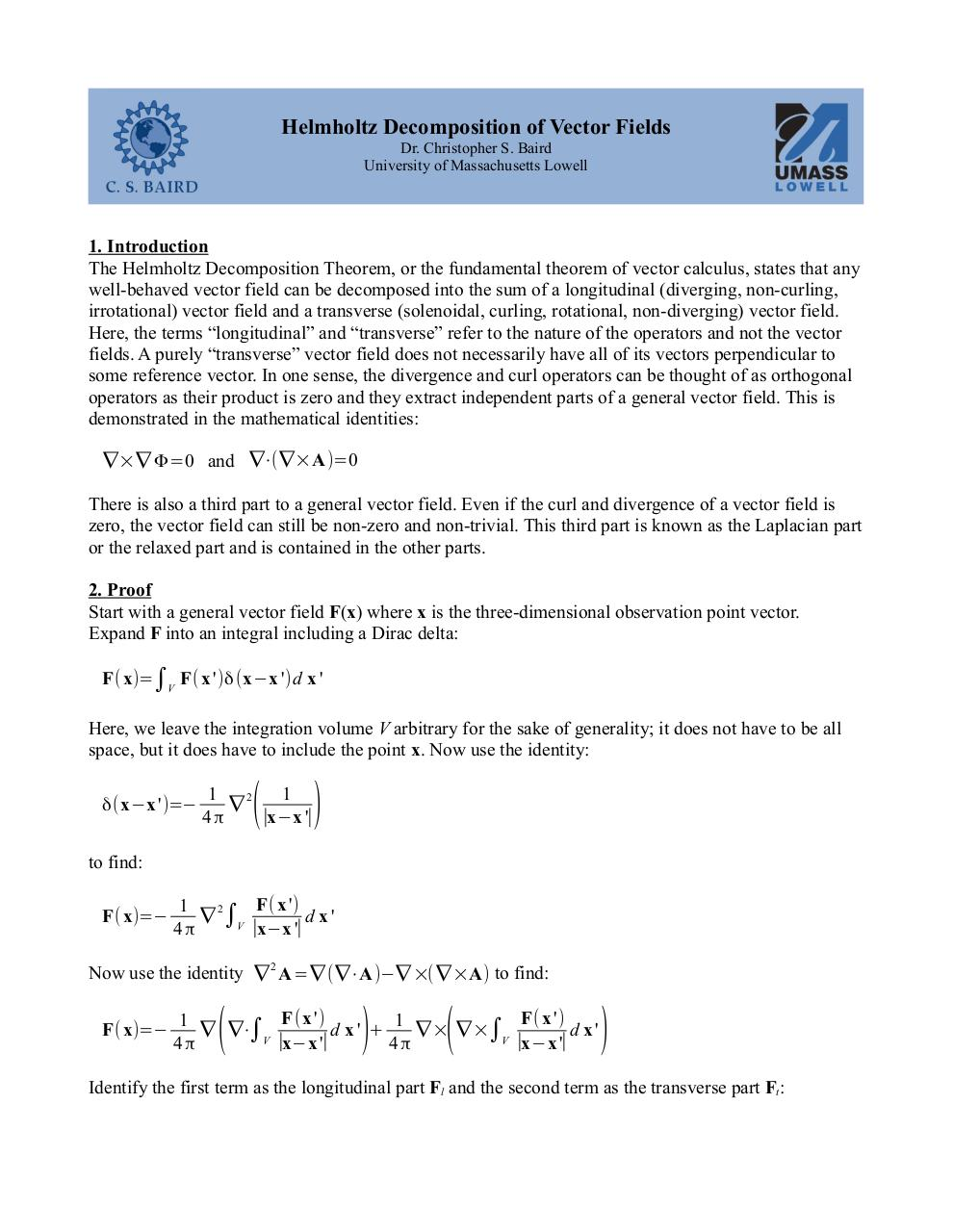# SuppHelmholtzDecomposition.pdfPage 1 2 3 4 5 6

#### Text preview

Helmholtz Decomposition of Vector Fields
Dr. Christopher S. Baird
University of Massachusetts Lowell

1. Introduction
The Helmholtz Decomposition Theorem, or the fundamental theorem of vector calculus, states that any
well-behaved vector field can be decomposed into the sum of a longitudinal (diverging, non-curling,
irrotational) vector field and a transverse (solenoidal, curling, rotational, non-diverging) vector field.
Here, the terms “longitudinal” and “transverse” refer to the nature of the operators and not the vector
fields. A purely “transverse” vector field does not necessarily have all of its vectors perpendicular to
some reference vector. In one sense, the divergence and curl operators can be thought of as orthogonal
operators as their product is zero and they extract independent parts of a general vector field. This is
demonstrated in the mathematical identities:
∇×∇ Φ=0 and ∇⋅(∇×A)=0
There is also a third part to a general vector field. Even if the curl and divergence of a vector field is
zero, the vector field can still be non-zero and non-trivial. This third part is known as the Laplacian part
or the relaxed part and is contained in the other parts.
2. Proof
Start with a general vector field F(x) where x is the three-dimensional observation point vector.
Expand F into an integral including a Dirac delta:
F( x)=∫V F(x ')δ (x−x ') d x '

Here, we leave the integration volume V arbitrary for the sake of generality; it does not have to be all
space, but it does have to include the point x. Now use the identity:
δ( x−x ')=−

(

)

1
1
∇2

∣x−x '∣

to find:
F( x)=−

F( x ')
1
2
∇ ∫V
d x'
∣x−x '∣

Now use the identity ∇ 2 A=∇ (∇⋅A)−∇ ×( ∇ ×A) to find:
F( x)=−

(

)

(

F( x ')
F( x ')
1
1
∇ ∇⋅∫V
d x' +
∇ × ∇ ×∫V
d x'
∣x−x '∣
∣x−x '∣

)

Identify the first term as the longitudinal part Fl and the second term as the transverse part Ft: